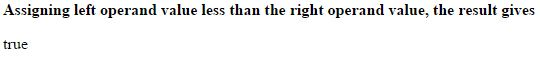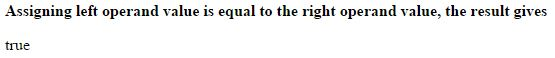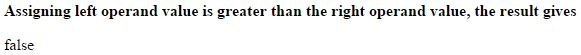# Less than or Equal to (<=) operator in JavaScript

### Less than or Equal to (<=)

Less than or Equal to operator is an Comparison Operator which is used to check the value of the left operand is either less than or equal to the value of the right operand.

If the value of the left operand is either less than or equal to the value of the right operand, the result gives 'true'.

The symbolic representation of Less than or Equal to is `<=`.

### Left operand value is less than right operand

```<b>Assigning left operand value less than the right operand value, the result gives</b>
<p id="myId"></p>

<script>
var a = 1;
var b = 3;
var c = (a <= b);
document.getElementById("myId").innerHTML = c;
</script>
```

In the above code snippet we assigned lesser value to the left operand and greater value to the right operand, we used less than or equal to operator, so the result gives 'true'.

OUTPUT### Left operand value is equal to right operand

```<b>Assigning left operand value is equal to the right operand value, the result gives</b>
<p id="myId"></p>

<script>
var a = 3;
var b = 3;
var c = (a <= b);
document.getElementById("myId").innerHTML = c;
</script>
```

Less than or Equal to with two equal values

In the above code snippet we assigned equal values to the left operand and right operand, so the result gives 'true'.

OUTPUT### Left operand value is greater than right operand

```<b>Assigning left operand value is greater than the right operand value, the result gives</b>
<p id="myId"></p>

<script>
var a = 3;
var b = 1;
var c = (a <= b);
document.getElementById("myId").innerHTML = c;
</script>```

Less than or Equal to with gretaer value at left operand

In the above code snippet we assigned greater value to the left operand and lesser value to the right operand, so the result gives 'false'.

OUTPUTViews: 5833 | Post Order: 174